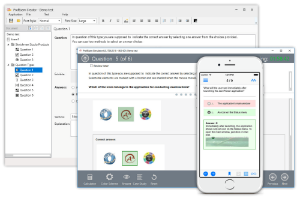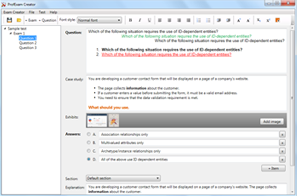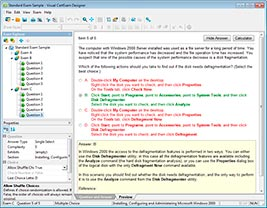File Info

 Exam Lean Six Sigma Green Belt Number LSSGB File Name Lean Six Sigma Green Belt.Testking.LSSGB.2018-11-09.1e.80q.vcex Size 35 Kb Posted November 09, 2018 Downloads 13

How to open VCEX & EXAM Files?

Files with VCEX & EXAM extensions can be opened by ProfExam Simulator.

Coupon: EXAMFILESCOM
With discount: 20%

Demo Questions

Question 1
A periodic time frame can be used to arrange for Control Limit and Center Line calculations with good SPC implementation in a process.

• A: True
• B: False

Question 2
The data on SPC charts are typically constructed such that they have the most recent data point on the right hand side.

• A: True
• B: False

Question 3
Which statement(s) describe an undesirable situation when implementing SPC?

• A: The lower Control Limit for the R chart is equal to zero
• B: Attempt to use SPC for tracking transaction times at a warehouse
• C: A process is in Statistical Control before implementation of SPC
• D: The Control Limits are wider than the customer specification limits

Question 4
If a process has Outliers which pair of charts is most preferable if subgroups will exist for the Continuous Data?

• A: Individual—Moving Range
• B: Xbar-R Charts
• C: Xbar-S Charts
• D: nP and P Charts

Question 5
After a Belt has put data through the smoothing process which chart would be used to look for trends in the data?

• A: Moving Average Chart
• B: Multi-Vari Chart
• C: X bar Chart
• D: Pareto Chart

Question 6
A Belt concludes a Lean Six Sigma project with the creation of a Control Plan. At what point can the Control Plan be closed?

• A: Never, a Control Plan is a living document
• B: As soon as the Champion signs off
• C: Within 30 days of the LSS project review team meeting
• D: After the project has been presented at the recognition event

Question 7
When analyzing a data set we frequently graph one metric as a function of another. If the slope of the Correlation line is -2.5 we would say the two metrics are ___________ correlated?

• A: Positively
• B: Not
• C: Negatively
• D: None

Question 8
Multiple Linear Regressions (MLR) is best used when which of these are applicable? (Note: There are 3 correct answers).

• A: Non-linear relationships between the inputs X’s and output Y
• B: Uncertainty in the slope of the linear relationship between an X and a Y
• C: Relationships between Y (output) and more than one X (Input)
• D: Preventing the use of a Designed Experiment if unnecessary
• E: We assume that the X’s are independent of each other

Question 9
Fractional Factorial designs for an experimental approach are used when ____________ about the multiple metric interaction in a process.

• A: Much is known
• B: Little is known
• C: We don’t care
• D: Data exists

Question 10
A Belt will occasionally do a quick experiment referred to as an OFAT which stands for ____________________.

• A: Only a Few Are Tested
• B: Opposite Factors Affect Technique
• C: One Factor At a Time
• D: Ordinary Fractional Approach Technique

CONNECT USPROFEXAM WITH A 20% DISCOUNT

You can buy ProfExam with a 20% discount..

HOW TO OPEN VCEX AND EXAM FILES

Use ProfExam Simulator to open VCEX and EXAM filesHOW TO OPEN VCE FILES

Use VCE Exam Simulator to open VCE files